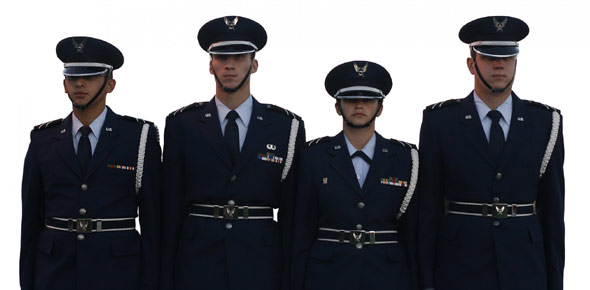# 2F071 CDC Review Exam Style

100 Questions | Total Attempts: 227SettingsReview of 2F071 CDC URE presented in Exam-Style

• 1.
What cleaning agent should be used to clean the probe on the conductivity meter?
• A.

Isopropyl alcohol.

• B.

Petroleum ether.

• C.

Turbine fuel.

• D.

Acetone.

• 2.
When should a refractometer used for fuel system icing inhibitor (FSII) tests be calibrated?
• A.

Prior to first use.

• B.

Weekly, and prior to first use.

• C.

Daily, or when changing test water.

• D.

Monthly, or when changing test water.

• 3.
What is the simplest and most expedient method of fuel resupply?
• A.

ABFDS

• B.

Ocean tanker

• C.

Aircraft systems

• D.

The same source of supply used by the host airfield

• 4.
When posting changes and supplements to publications, within what maximum number of days of receipt should you file technical order (TO) changes and supplements?
• A.

1.

• B.

5.

• C.

7.

• D.

10.

• 5.
What is a major difference between a gas and a liquid?
• A.

A liquid is far less compressible than a gas.

• B.

A gas is far less compressible than a liquid.

• C.

A liquid is more combustible than a gas.

• D.

A gas is more combustible than a liquid.

• 6.
What is the lifting force of a liquid called?
• A.

Laminar.

• B.

Interface.

• C.

Buoyance.

• D.

Dynamic pressure.

• 7.
Which best describes immiscible liquids?
• A.

They mix well together.

• B.

One liquid will float on the other.

• C.

They will become an intrained solution.

• D.

They will repel each other and react violently.

• 8.
Which unit of measurement would indicate velocity of flow?
• A.

Feet per second

• B.

Gallons per minute.

• C.

Cubic feet per second.

• D.

Cubic gallons per minute.

• 9.
Which physical factor affects the behavior of liquids in both rest and motion?
• A.

Friction, applied forces, and gravity.

• B.

Friction, inertia, and atmospheric pressure.

• C.

Gravity, atmospheric pressure, and inertia.

• D.

Gravity, atmospheric pressure, and applied forces.

• 10.
What determines the intensity of the excess pressure in a water-hammer wave?
• A.

The size of the pipe.

• B.

The specific gravity of the fuel.

• C.

The change in gallons per minute.

• D.

The amount of extinguished velocity.

• 11.
Which type of measurement may be used to express pressure?
• A.

Kilograms.

• B.

Pounds.

• C.

Tons.

• D.

Pounds per square inch.

• 12.
The standard measure for atmospheric pressure is equal to how many pounds per square inch?
• A.

13.1 psi.

• B.

14.7 psi.

• C.

15.2 psi.

• D.

17.9 psi.

• 13.
What type of pressure reading is also referred to as overpressure?
• A.

Zero pressure.

• B.

Gauge pressure.

• C.

Absolute pressure.

• D.

Atmospheric pressure.

• 14.
What term is used to describe the total pressure acting as a gas?
• A.

Overpressure.

• B.

Gauge pressure.

• C.

Absolute pressure.

• D.

Atmospheric pressure.

• 15.
Which temperature scale is commonly used for scientific measurements?
• A.

Kelvin scale.

• B.

Rankine scale.

• C.

Réaumur scale.

• D.

Fahrenheit scale.

• 16.
Which gas law declares that cooling a gas decreases its volume?
• A.

Boyle’s law.

• B.

Charles’ law.

• C.

Dalton’s law.

• D.

Gay-Lussac’s law.

• 17.
Two or more atoms combined is called a
• A.

A compound.

• B.

An element.

• C.

A molecule.

• D.

A mixture.

• 18.
According to the electron theory, atoms are composed of
• A.

Protons, neutrons, and electrons.

• B.

Neutrons, elements, and protons.

• C.

Electrons, molecules, and elements.

• D.

Electrons, neutrons, and matter.

• 19.
How do the charges of protons and electrons differ?
• A.

Protons have positive charges, and electrons have neutral charges.

• B.

Protons have neutral charges, and electrons have positive charges.

• C.

Protons have negative charges, and electrons have positive charges.

• D.

Protons have positive charges, and electrons have negative charges.

• 20.
The uniform movement of electrons in a specific direction is called
• A.

Electrical potential.

• B.

Free electrons.

• C.

Electric current.

• D.

Ionization.

• 21.
Which shell of the valence electrons determines whether a material is a good or a poor conductor of electricity?
• A.

The outermost shell.

• B.

The middle shell.

• C.

The second shell.

• D.

The first shell.

• 22.
Which statement best describes a positive ion?
• A.

An atom that lost a proton and has more electrons.

• B.

An atom that lost an electron and has more protons.

• C.

An atom that has an equal number of electrons and protons.

• D.

An atom that cannot throw off any protons.

• 23.
What effect of current causes an electric motor to produce mechanical energy?
• A.

Heat.

• B.

Magnetism.

• C.

Chemical.

• D.

Reactance.

• 24.
What is the unit of measurement for electrical power?
• A.

Watts.

• B.

Volts.

• C.

Amps.

• D.

Ohms.

• 25.
A 3-horsepower motor consumes how much power?
• A.

755 W.

• B.

1,534 W.

• C.

2,023 W.

• D.

2,238 W.

Related TopicsBack to top